# 聚合查询 aggregate

## 聚合函数

`Max Min Sum Avg Count`

• `aggregate`,

• 需要导入模块：`from django.db.models import Max, Min, Sum, Avg, Count`

``````# 1.筛选出价格最高的书籍
from django.db.models import Max, Min, Sum, Avg, Count
res = models.Book.objects.aggregate(max=Max('price'))
print(res)
# 2.求书籍总价格
res = models.Book.objects.aggregate(sum=Sum('price'))
print(res)
# 3.求书籍平均价格
res = models.Book.objects.aggregate(avg=Avg('price'))
print(res)

# 4.一起使用
res = models.Book.objects.aggregate(Max('price'),Min('price'),Sum('price'),Count('price'),Avg('price'))
print(res)``````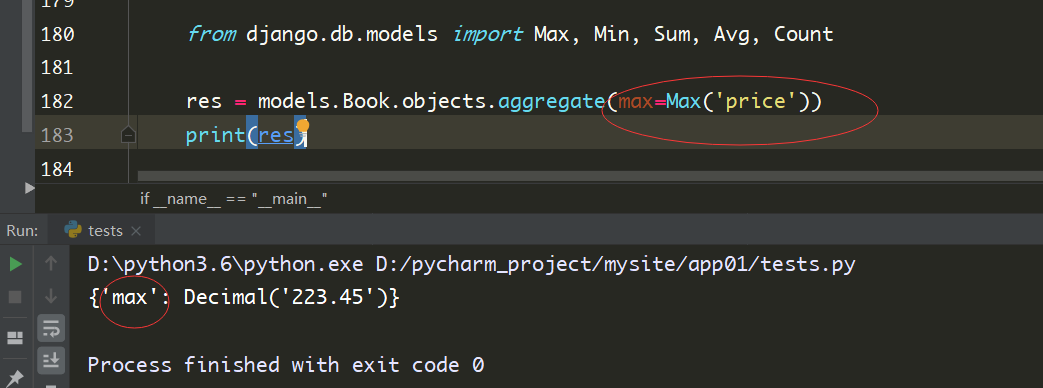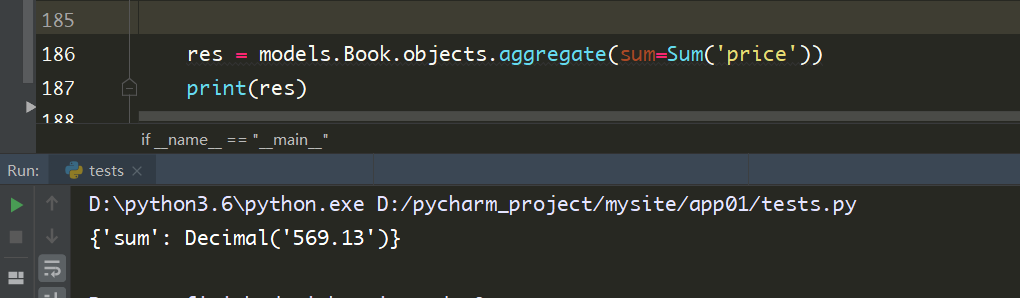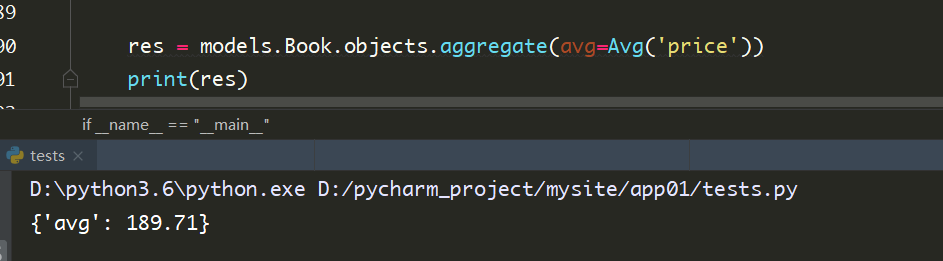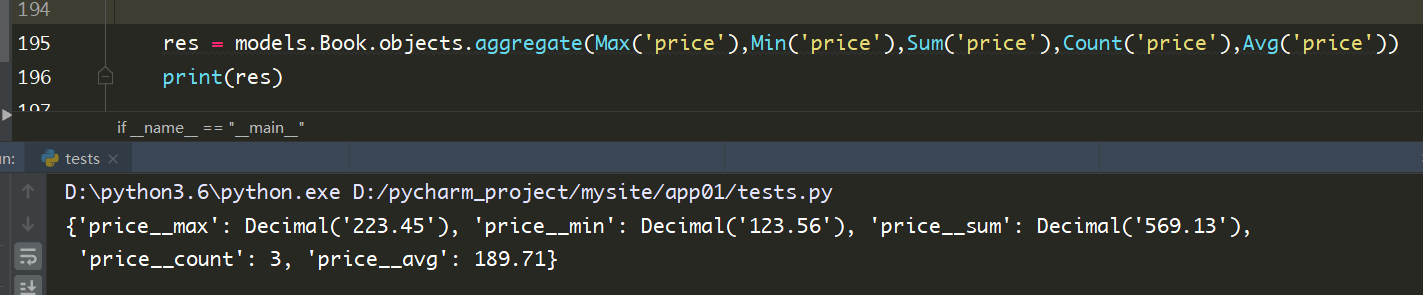# 分组查询 annotate

``````# 1.统计每一本书的书名 和对应的作者人数
res = models.Book.objects.annotate(author_num=Count('authors__pk')).values('title', 'author_num')
print(res)
# 2.统计出每个出版社卖的最便宜的书的价格  出版社的名字 价格
res = models.Publish.objects.annotate(min_price=Min('book__pk')).values('name', 'min_price')
print(res)
# 3.统计不止一个作者的图书
res = models.Book.objects.annotate(author_num=Count('authors')).filter(author_num__gt=1).values('title', 'author_num')
print(res)
# 4.查询各个作者出的书的总价格  作者名字  总价格
res = models.Author.objects.annotate(sum_price=Sum('book__price')).values('name', 'sum_price')
print(res)``````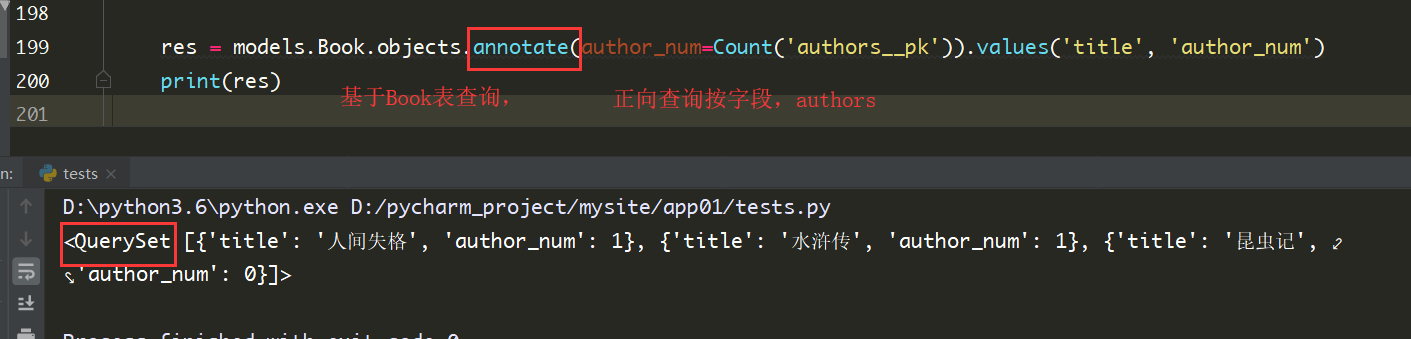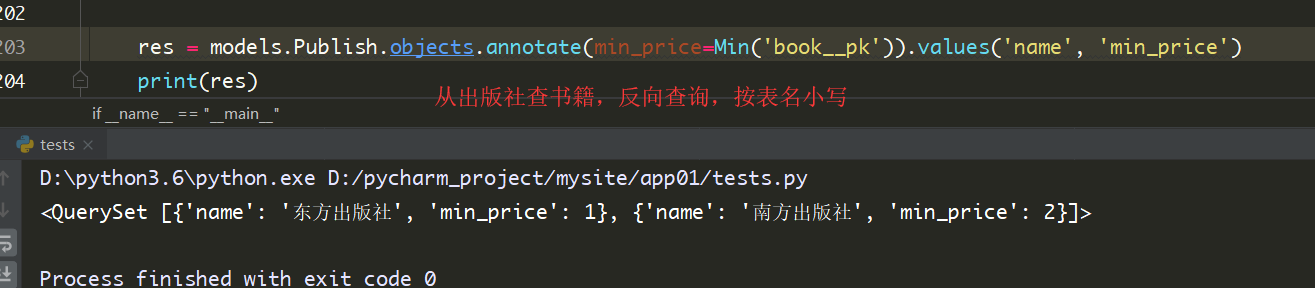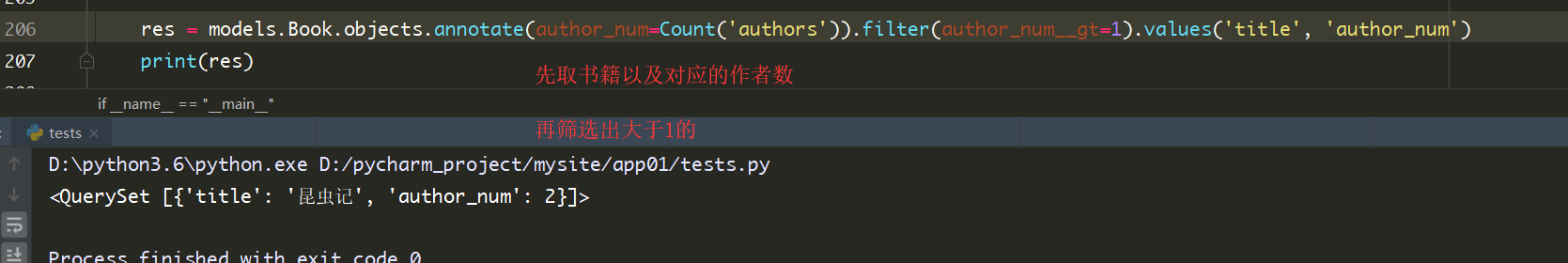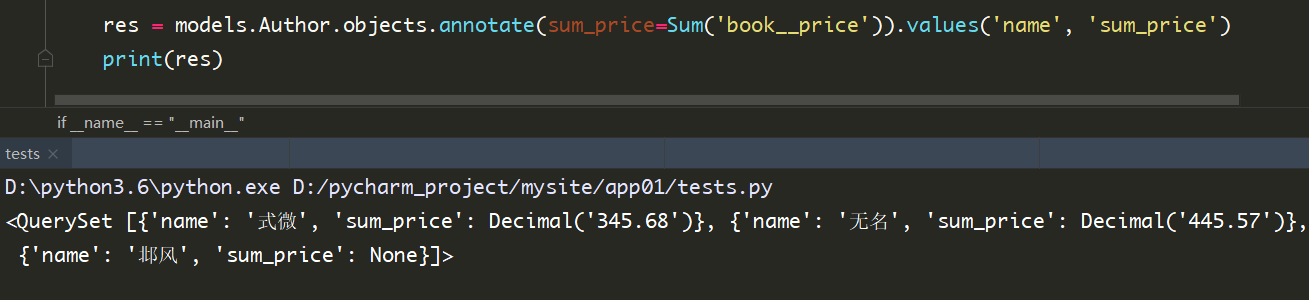# F与Q查询

## F查询

1. 查询卖出数(sold)大于库存(stock)的商品
``````from django.db.models import Q
res = models.Book.objects.filter(sold__gt=F('stock')).values('title')
print(res)``````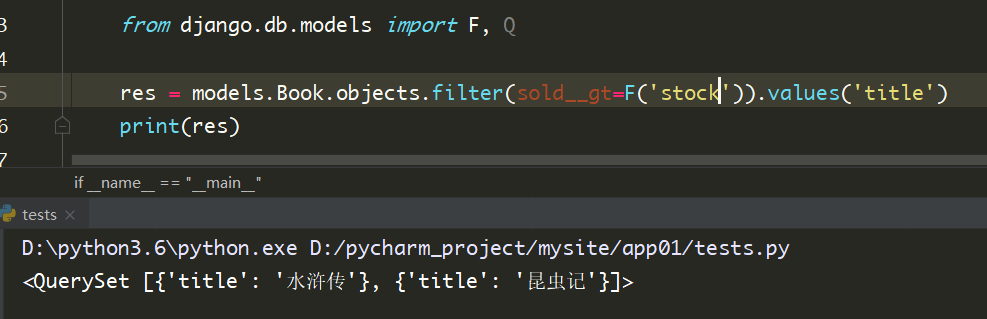1. 将所有书的价格上涨100块
``models.Book.objects.all().update(price=F('price') + 100)``
1. 将所有书的名称后面全部加上 “新款” 后缀
``````from django.db.models.functions import Concat
from django.db.models import Value
models.Book.objects.update(title=Concat(F('title'), Value('新款')))``````

## Q查询

`filter()` 等方法中逗号隔开的条件是and的关系。 如果你需要执行更复杂的查询，如or关系，需要借助`Q()`

``````from django.db.models import Q
# res = models.Book.objects.filter(Q(title='昆虫记'), Q(stock=500))   # 这样使用逗号还是and关系
res = models.Book.objects.filter(Q(title='昆虫记') | Q(stock=500))   # 使用 | 变成 or关系``````

Q对象高级用法

``````from django.db.models import Q
q = Q()
q.connector = 'or'   # 默认是and关系，这里指定or关系
q.children.append(('title', '昆虫记'))   # 这里是元组
q.children.append(('stock__gt', 500))
res = models.Book.objects.filter(q)
print(res)``````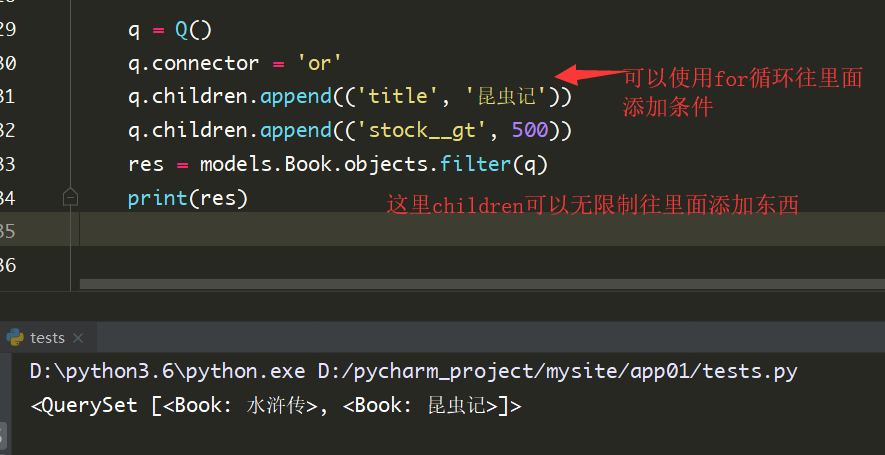# ORM操作事务

• 原子性：事务的操作是一个整体，不可分割，是最小单位
• 一致性：数据库总是从一个一致性的状态转换到另一个一致性的状态。因为事务最终没有提交，所以事务中所做的修改也不会保存到数据库中。
• 隔离性：事务与事务的操作是隔离的
• 一旦事务提交，则其所做的修改会永久保存到数据库，不可修改

• 第一范式是最基本的范式，如果数据库表中的所有字段值都是不可分解的原子值，说明就满足的第一范式
• 第二范式是在第一范式的基础上的，另外包含两部分内容，一是表必须有一个主键；二是没有包含在主键中的列必须完全依赖于主键，而不能只依赖主键的一部分。也就是说在一个数据表中，只能保存一种数据，不能把多种数据保存在同一张表中。
• 第三范式基于第二范式， 确保数据表中每一列数据都和主键直接相关，而不能间接相关。要求一个关系中不包含在其他关系已包含的非主键字段信息。

## django中开启事务

``````from django.db import transaction
with transaction.atomic():
# 在缩进的代码中书写数据库操作
# 该缩进内的所有代码， 都是一个事务
pass
# 事务在with外自动结束``````

https://www.cnblogs.com/setcreed/p/11953663.html

「点点赞赏，手留余香」

还没有人赞赏，快来当第一个赞赏的人吧！
Python
Python
0 条回复 A 作者 M 管理员
所有的伟大，都源于一个勇敢的开始！